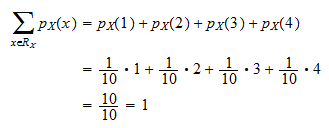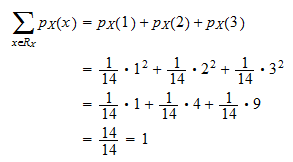StatLect

Legitimate probability mass functions

In this lecture we analyze two properties of probability mass functions. We prove not only that any probability mass function satisfies these two properties, but also that any function satisfying these two properties is a legitimate probability mass function.Properties of probability mass functions

Any probability mass function satisfies two basic properties, as shown by the next proposition.

Proposition (Properties of a probability mass function) Letbe a discrete random variable and letbe its probability mass function. The probability mass functionsatisfies the following two properties:

1. Non-negativity:for any;

2. Sum over the support equals:, whereis the support of.

Proof

Remember that, by the definition of a probability mass function,is such thatProbabilities cannot be negative, thereforeand, as a consequence,. This proves property 1 above (non-negativity).

Furthermore, the probability of a sure thing must be equal to. Since, by the very definition of support, the eventis a sure thing, thenwhich proves property 2 above (sum over the support equals).

Identification of legitimate probability mass function

Any probability mass function must satisfy property 1 and 2 above. Using some standard results from measure theory (omitted here), it is possible to prove that the converse is also true, that is, any functionsatisfying the two properties above is a probability mass function.

Proposition (Legitimate probability mass function) Letbe a function satisfying the following two properties:

1. Non-negativity:for any;

2. Sum over the support equals:, whereis the support of.

Then, there exists a discrete random variablewhose probability mass function is.

This proposition gives us a powerful method for constructing probability mass functions. Take a subset of the set of real numbers. Take any functionthat is non-negative on(non-negative means thatfor any). If the sumis well-defined and is finite and strictly positive, then defineis strictly positive, thusis non-negative and it satisfies property 1. It also satisfies Property 2 becauseTherefore, any functionthat is non-negative on(is chosen arbitrarily) can be used to construct a probability mass function if its sum overis well-defined and is finite and strictly positive.

Example Defineand a functionas follows:Can we useto build a probability mass function? First of all, we have to check thatis non-negative. This is obviously true, becauseis always non-negative. Then, we have to check that the sum ofoverexists and is finite and strictly positive:Sinceexists and is finite and strictly positive, we can defineBy the above proposition,is a legitimate probability mass function.

Solved exercises

Below you can find some exercises with explained solutions.

Exercise 1

Consider the following function:Prove thatis a legitimate probability mass function.

Solution

Forwe havewhile forwe haveTherefore,for anyand the non-negativity property is satisfied. The other necessary property (sum over the support equals) is also satisfied becauseExercise 2

Consider the following function:Prove thatis a legitimate probability mass function.

Solution

Forwe havewhile forwe haveTherefore,for anyand the non-negativity property is satisfied. The other necessary property (sum over the support equals) is also satisfied becauseExercise 3

Consider the following function:Prove thatis a legitimate probability mass function.

Solution

Forwe havebecauseis strictly positive. Forwe haveTherefore,for anyand the non-negativity property is satisfied. The other necessary property (sum over the support equals) is also satisfied becauseThe book

Most of the learning materials found on this website are now available in a traditional textbook format.

Glossary entries
Share